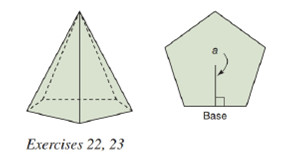Chapter 9.2, Problem 23EElementary Geometry For College St...

7th Edition
Alexander + 2 others
ISBN: 9781337614085

Solutions

Chapter
SectionElementary Geometry For College St...

7th Edition
Alexander + 2 others
ISBN: 9781337614085
Textbook Problem

In a regular pentagonal pyramid, each base edge measures 9.2 cm and the apothem of the base measures 6.3 cm . The altitude of the pyramid measures 14.6 cm .a) Find the base area of the pyramid.b) Find the volume of the pyramid.To determine

(a)

To find:

The base area of the pyramid.

Explanation

Given:

A regular pentagonal pyramid, each base edge measures 9.2cm and the apothem of the base measures 6.3cm and the altitude of the pyramid measures 14.6cm.

Properties used:

A pyramid is made by connecting a base to an apex. The base is flat with straight edges, no curves, hence, a polygon and all other faces are triangles.

A regular pyramid is a pyramid whose base is a regular polygon and whose lateral edges are all congruent.

The base area B of a regular pyramid is given by the area of the polyhedron formed.

Calculation:

From the given figure,

Each base edge measures 9.2cm and the apothem of the base measures 6

To determine

(b)

To find:

The volume of the pyramid.

Still sussing out bartleby?

Check out a sample textbook solution.

See a sample solution

The Solution to Your Study Problems

Bartleby provides explanations to thousands of textbook problems written by our experts, many with advanced degrees!

Get Started

Find more solutions based on key concepts